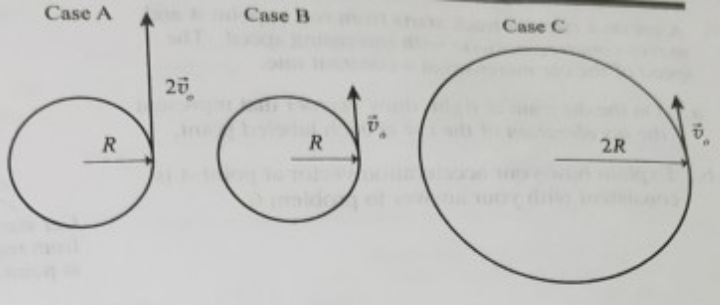# Problem: Three cars move at constant speed around three circular tracks as shown in the top-view diagram at right.In cases A and B, the radius of the track is R: in case C, 2R. In case A, the speed of the car is 2vo; in case B and C, vo.a. Rank the three cases according to the magnitude of the acceleration, from largest to smallest. If the magnitude of the acceleration is the same in any of the cases, state so explicitly. Explain your reasoning using the general rules you devised in tutorial.b. Explain how your ranking above is consistent with the result for the magnitude of the acceleration for the case of uniform circular motion: a = v2/r, where v is the speed of the object and r is the radius of the circular trajectory.

###### FREE Expert Solution

a.

All the cars are moving at a constant speed.

Therefore, the tangential acceleration is zero.

What remains is only radial acceleration.

96% (60 ratings)###### Problem Details

Three cars move at constant speed around three circular tracks as shown in the top-view diagram at right.

In cases A and B, the radius of the track is R: in case C, 2R. In case A, the speed of the car is 2vo; in case B and C, vo.a. Rank the three cases according to the magnitude of the acceleration, from largest to smallest. If the magnitude of the acceleration is the same in any of the cases, state so explicitly. Explain your reasoning using the general rules you devised in tutorial.

b. Explain how your ranking above is consistent with the result for the magnitude of the acceleration for the case of uniform circular motion: a = v2/r, where v is the speed of the object and r is the radius of the circular trajectory.